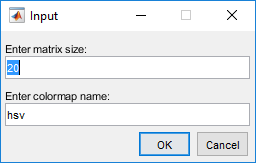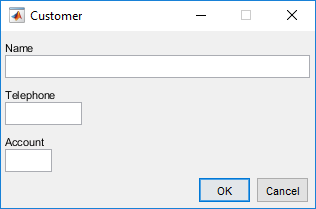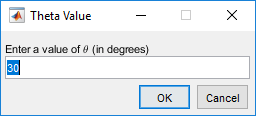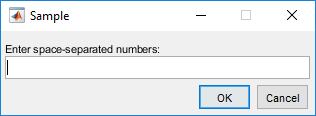# inputdlg

Create dialog box to gather user input

## Syntax

answer = inputdlg(prompt)
answer = inputdlg(prompt,dlgtitle)
answer = inputdlg(prompt,dlgtitle,dims)
answer = inputdlg(prompt,dlgtitle,dims,definput)
answer = inputdlg(prompt,dlgtitle,dims,definput,opts)

## Description

example

answer = inputdlg(prompt) creates a modal dialog box containing one or more text edit fields and returns the values entered by the user. The return values are elements of a cell array of character vectors. The first element of the cell array corresponds to the response in the edit field at the top of the dialog box. The second element corresponds to the next edit field response, and so on. 

example

answer = inputdlg(prompt,dlgtitle) specifies a title for the dialog box.

example

answer = inputdlg(prompt,dlgtitle,dims) specifies the height of each edit field when dims is a scalar value. When dims is an array, the first value in each array element sets the edit field height. The second value in each array element sets the edit field width.

example

answer = inputdlg(prompt,dlgtitle,dims,definput) specifies the default value for each edit field. The definput input argument must contain the same number of elements as prompt.

example

answer = inputdlg(prompt,dlgtitle,dims,definput,opts)specifies that the dialog box is resizeable in the horizontal direction when opts is set to 'on'. When opts is a structure, it specifies whether the dialog box is resizeable in the horizontal direction, whether it is modal, and whether the prompt text is interpreted.

## Examples

collapse all

Create a dialog box that contains two text edit fields to get integer and colormap name inputs from users.

prompt = {'Enter matrix size:','Enter colormap name:'}; dlgtitle = 'Input'; dims = [1 35]; definput = {'20','hsv'}; answer = inputdlg(prompt,dlgtitle,dims,definput)Create an input dialog box titled Customer that contains three edit fields of different widths.

x = inputdlg({'Name','Telephone','Account'},... 'Customer', [1 50; 1 12; 1 7]);Create a dialog box that uses the options structure to render the prompt using TeX markup.

Define the options for the dialog box by specifying:

• A prompt that includes the \theta character sequence

• A dialog title

• A default input value of 30

• An edit field height equal to 1 row of text and width equal to 40 character units

• TeX as the interpreter

Then, create the input dialog by specifying these options.

prompt = {'Enter a value of \theta (in degrees)'}; dlgtitle = 'Theta Value'; dims = [1 40]; definput = {'30'}; opts.Interpreter = 'tex'; answer = inputdlg(prompt,dlgtitle,dims,definput,opts);Users can enter scalar or vector values into inputdlg text edit fields. MATLAB® stores the input as a cell array of character vectors. Convert a member of the input cell array to a number, using str2num.

Create an input dialog box that asks users to enter numerical data.

answer = inputdlg('Enter space-separated numbers:',... 'Sample', [1 50])Suppose that the user enters 1 2 3 4 -5 6+7, and then clicks . MATLAB stores the answer as a cell array of character vectors – {'1 2 3 4 -5 6+7'}

Use str2num to convert the cell array to numerals.

user_val = str2num(answer{1}) 
user_val = 1 2 3 4 -5 13

## Input Arguments

collapse all

Text edit field labels, specified as a character vector, cell array of character vectors, or string array. For cell arrays and string arrays, each element specifies an edit field label. Both types of arrays specify a top to bottom sequence of edit fields in the dialog box.

Example: {'First Name','Last Name'}

Dialog box title, specified as a character vector or string scalar.

Example: 'Data'

Text edit field height and width (dimensions), specified as one of these values:

• If dims is a scalar, then it specifies the height for all edit fields. Height is the distance between the baselines of two lines of text. The width for all edit fields is the maximum that the dialog box allows.

• If dims is a column vector or row vector, then each element specifies the height of each corresponding edit field from the top to the bottom of the dialog box. The width for all edit fields is the maximum that the dialog box allows.

• If dims is an array, then it must be size m-by-2, where m is the number of prompts in the dialog box. Each row refers to the edit field for the corresponding prompt. The first column specifies the height of the edit field in lines of text. The second column specifies the width of that edit field in character units. The width of a character unit equals the width of the letter x when using the system font.

Note

With one exception, the height and width of the edit fields do not limit the amount of text that users can enter. When the edit field height is 1, users cannot enter multiple lines of text.

Example: 2 specifies that the height of each edit field in the dialog box is two lines of text.

Example: [1;2] specifies that the height of the first (top-most) edit field is one line of text and the height of the second edit field is two lines of text.

Example: [1 50; 2 10] specifies that the first (top-most) edit field is one line high and 50 character units wide and that the second edit field is two lines high and 10 character units wide.

Default input value or values, specified as a cell array of character vectors or string array.

Example: {'Color','1'}

Dialog box settings specified as 'on' or a structure. When opts is set to 'on', then users can resize the dialog box in the horizontal direction. When opts is a structure, the structure fields specify the options in the following table.

Field

Values

Resize

'off' (default) or 'on'. If set to 'off', then users cannot resize the dialog box. If 'on', then users can resize the window horizontally.

WindowStyle

'modal' (default) or 'normal'. If set to 'modal', then users cannot interact with other windows before responding.

Interpreter

'none' (default) or 'tex'. If set to 'tex', then the prompt is rendered using TeX. The dialog box title is not affected.

Use TeX markup to add superscripts and subscripts, modify the font type and color, and include special characters in the prompt text.

Modifiers remain in effect until the end of the text. Superscripts and subscripts are an exception because they modify only the next character or the characters within the curly braces. When you set the interpreter to 'tex', the supported modifiers are as follows.

ModifierDescriptionExample
^{ }Superscript'text^{superscript}'
_{ }Subscript'text_{subscript}'
\bfBold font'\bf text'
\itItalic font'\it text'
\slOblique font (usually the same as italic font)'\sl text'
\rmNormal font'\rm text'
\fontname{specifier}Font name — Replace specifier with the name of a font family. You can use this in combination with other modifiers.'\fontname{Courier} text'
\fontsize{specifier}Font size —Replace specifier with a numeric scalar value in point units.'\fontsize{15} text'
\color{specifier}Font color — Replace specifier with one of these colors: red, green, yellow, magenta, blue, black, white, gray, darkGreen, orange, or lightBlue.'\color{magenta} text'
\color[rgb]{specifier}Custom font color — Replace specifier with a three-element RGB triplet.'\color[rgb]{0,0.5,0.5} text'

This table lists the supported special characters for the 'tex' interpreter.

Character SequenceSymbolCharacter SequenceSymbolCharacter SequenceSymbol

\alpha

α

\upsilon

υ

\sim

~

\angle

\phi

ϕ

\leq

\ast

*

\chi

χ

\infty

\beta

β

\psi

ψ

\clubsuit

\gamma

γ

\omega

ω

\diamondsuit

\delta

δ

\Gamma

Γ

\heartsuit

\epsilon

ϵ

\Delta

Δ

\spadesuit

\zeta

ζ

\Theta

Θ

\leftrightarrow

\eta

η

\Lambda

Λ

\leftarrow

\theta

θ

\Xi

Ξ

\Leftarrow

\vartheta

ϑ

\Pi

Π

\uparrow

\iota

ι

\Sigma

Σ

\rightarrow

\kappa

κ

\Upsilon

ϒ

\Rightarrow

\lambda

λ

\Phi

Φ

\downarrow

\mu

µ

\Psi

Ψ

\circ

º

\nu

ν

\Omega

Ω

\pm

±

\xi

ξ

\forall

\geq

\pi

π

\exists

\propto

\rho

ρ

\ni

\partial

\sigma

σ

\cong

\bullet

\varsigma

ς

\approx

\div

÷

\tau

τ

\Re

\neq

\equiv

\oplus

\aleph

\Im

\cup

\wp

\otimes

\subseteq

\oslash

\cap

\in

\supseteq

\supset

\lceil

\subset

\int

\cdot

·

\o

ο

\rfloor

\neg

¬

\nabla

\lfloor

\times

x

\ldots

...

\perp

\surd

\prime

´

\wedge

\varpi

ϖ

\0

\rceil

\rangle

\mid

|

\vee

\langle

\copyright

Example: 'on'

Example: opts.Resize = 'on'

Example: opts.WindowStyle = 'normal'

Example: opts.Interpreter = 'tex'

## Output Arguments

collapse all

Returns a cell array of character vectors containing one input per edit field, starting from the top of the dialog box. Use the str2num function to convert space-delimited and comma-delimited values into row vectors, and semicolon-delimited values into column vectors. For an example, see Convert Input to Numeric Values.

If the user clicks the button to close the dialog box, then answer is an empty cell array, { }.

If the user presses the keyboard Return key to close the dialog box, then answer is the value of definput. If definput is undefined, then answer is an empty cell array, { }.

collapse all

### Modal Dialog Box

A modal dialog box prevents a user from interacting with other MATLAB windows before responding to the dialog box.

## Tips

• MATLAB program execution continues even when a modal input dialog box is active. To block program execution until the user responds, use the uiwait function.

Users can enter scalar or vector values into Input dialog boxes. Use str2num to convert space-delimited and comma-delimited values into row vectors and to convert semicolon-delimited values into column vectors. For example, if answer{1} contains '1 2 3;4 -5 6+7i', the conversion produces:

input = str2num(answer{1}) input = 1.0000 2.0000 3.0000 4.0000 -5.0000 6.0000 + 7.0000i

## Version History

Introduced before R2006a